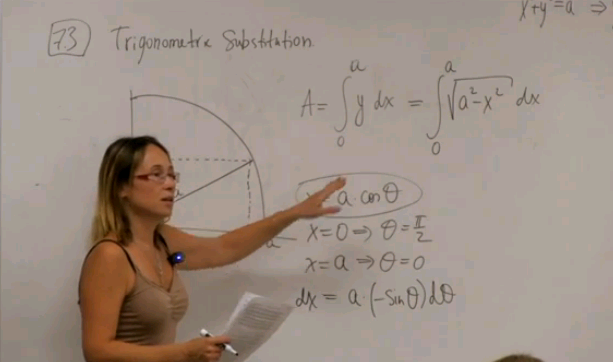# Home

## Math 2B. Lec. 12. Calculus: Trigonometric Substitution (English)

UCI Math 2B: Single-Variable Calculus (Fall 2013)
Lec 12. Single-Variable Calculus -- Trigonometric Substitution --
View the complete course: http://ocw.uci.edu/courses/math_2b_calculus.html
Instructor: Natalia L. Komarova Ph.D.

More courses at http://ocw.uci.edu

Description: UCI Math 2B is the second quarter of Single-Variable Calculus and covers the following topics: Definite integrals; the fundamental theorem of calculus. Applications of integration including finding areas and volumes. Techniques of integration. Infinite sequences and series. Parametric and polar equations.

Recorded on October 25, 2013

Required attribution: Komarova, Natalia L. Math 2B (UCI OpenCourseWare: University of California, Irvine), http://ocw.uci.edu/courses/math_2b_calculus.html. [Access date]. License: Creative Commons Attribution-ShareAlike 3.0 United States License.Author:
Natalia Komarova
Title:
Professor
Department:
Mathematics